# Data visualisation Olympics - Visualization in R

Overview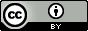Questions:
• How does plotting work in R?

• How can I facet plots?

• How do I produce a nice, publication ready plot with ggplot2?

Objectives:
• Produce scatter plots, boxplots, and time series plots using ggplot.

• Set universal plot settings.

• Describe what faceting is and apply faceting in ggplot.

• Modify the aesthetics of an existing ggplot plot (including axis labels and color).

• Build complex and customized plots from data in a data frame.

Time estimation: 1 hour
Level: Introductory Introductory
Supporting Materials:
Last modification: Oct 23, 2023
purl PURL: https://gxy.io/GTN:T00353
version Revision: 10
Best viewed in RStudio

This tutorial is available as an RMarkdown file and best viewed in RStudio! You can load this notebook in RStudio on one of the UseGalaxy.* servers

Launching the notebook in RStudio in Galaxy

1. Instructions to Launch RStudio
2. Access the R console in RStudio (bottom left quarter of the screen)
3. Run the following code:
``````download.file("https://training.galaxyproject.org/training-material/topics/data-science/tutorials/data-manipulation-olympics-viz-r/data-science-data-manipulation-olympics-viz-r.Rmd", "data-science-data-manipulation-olympics-viz-r.Rmd")
``````
4. Double click the RMarkdown document that appears in the list of files on the right.

1. Right click this link: tutorial.Rmd

Alternative Formats

1. This tutorial is also available as a Jupyter Notebook (With Solutions), Jupyter Notebook (Without Solutions)
Hands-on: Learning with RMarkdown in RStudio

Learning with RMarkdown is a bit different than you might be used to. Instead of copying and pasting code from the GTN into a document you’ll instead be able to run the code directly as it was written, inside RStudio! You can now focus just on the code and reading within RStudio.

1. Load the notebook if you have not already, following the tip box at the top of the tutorial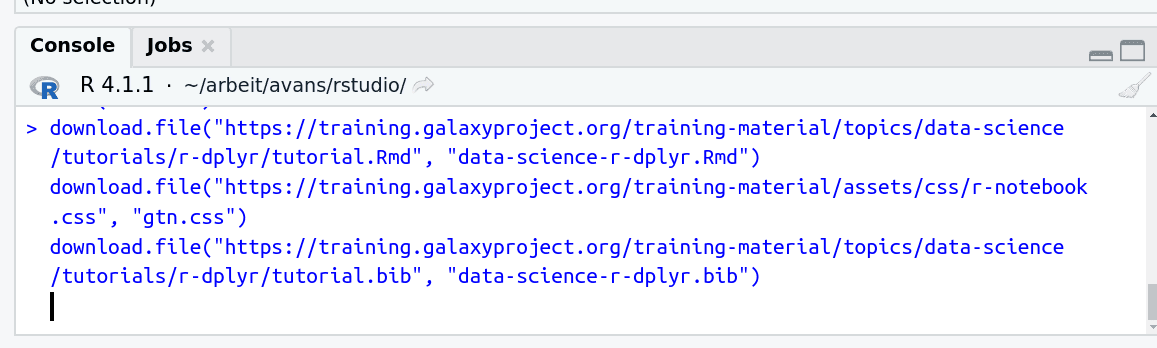2. Open it by clicking on the `.Rmd` file in the file browser (bottom right)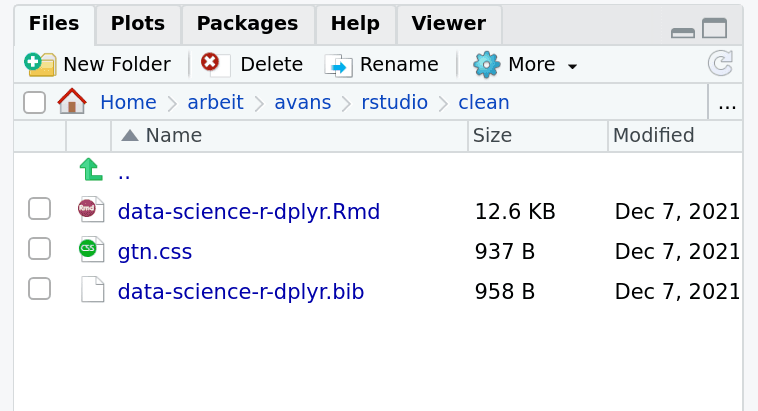3. The RMarkdown document will appear in the document viewer (top left)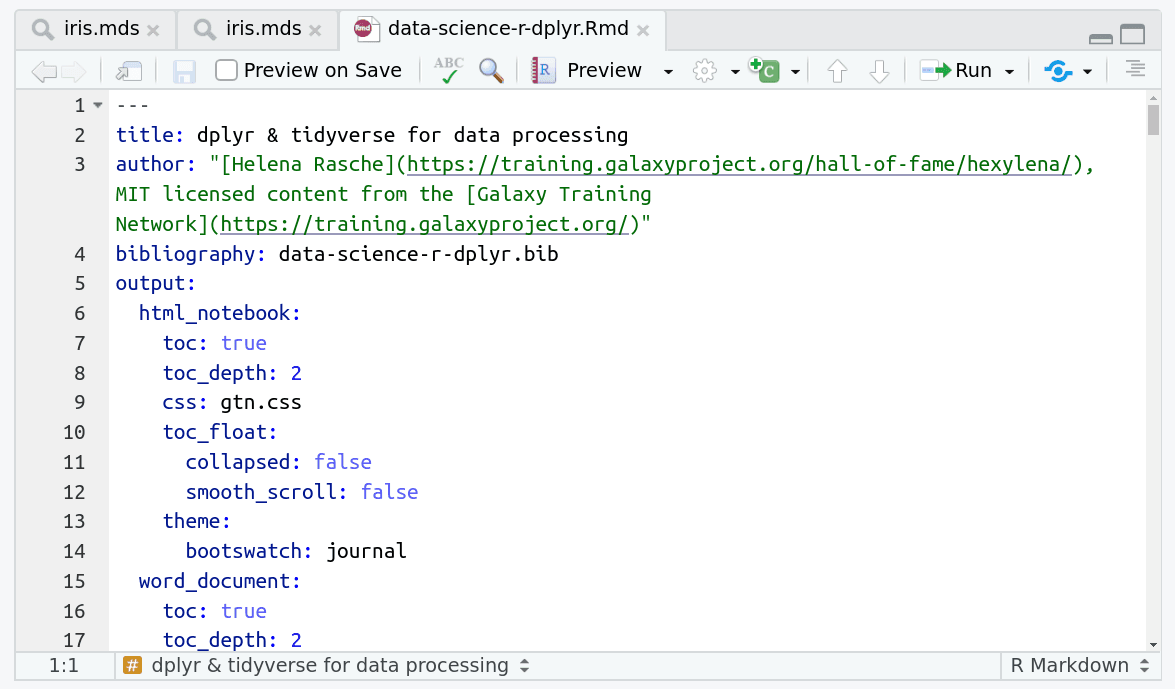You’re now ready to view the RMarkdown notebook! Each notebook starts with a lot of metadata about how to build the notebook for viewing, but you can ignore this for now and scroll down to the content of the tutorial.

You can switch to the visual mode which is way easier to read - just click on the gear icon and select `Use Visual Editor`.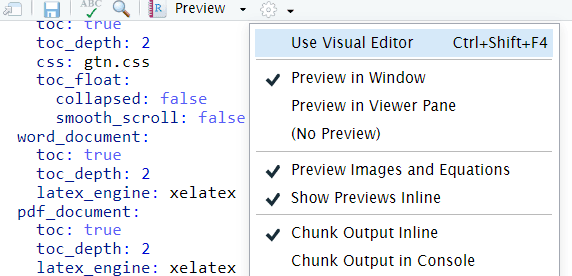You’ll see codeblocks scattered throughout the text, and these are all runnable snippets that appear like this in the document: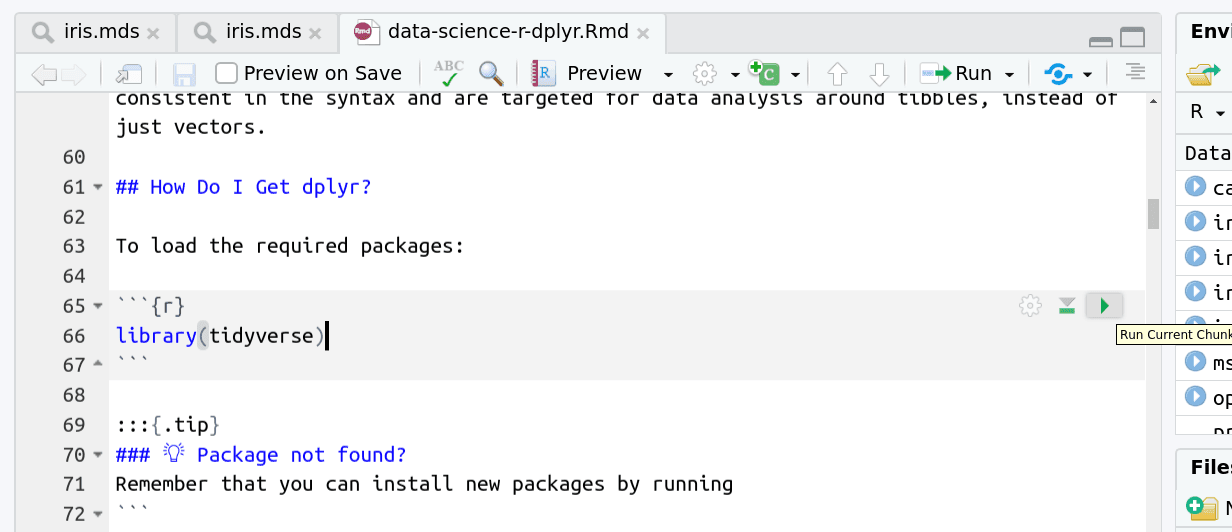And you have a few options for how to run them:

1. Click the green arrow
2. ctrl+enter
3. Using the menu at the top to run all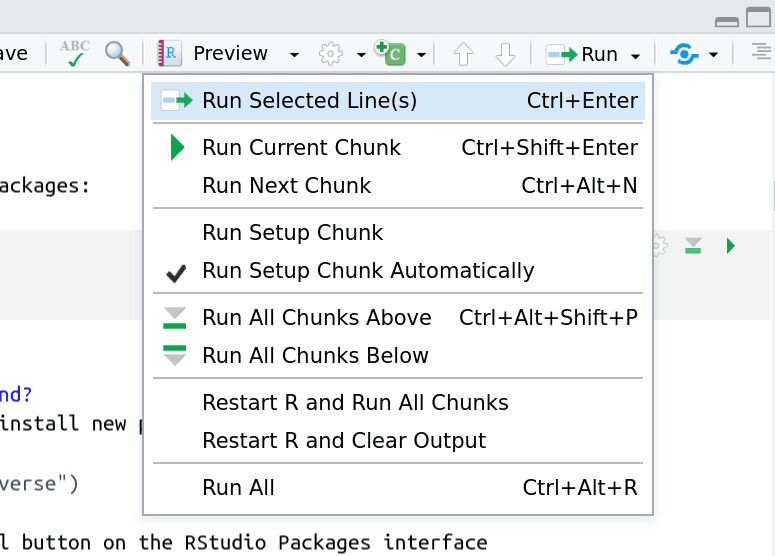When you run cells, the output will appear below in the Console. RStudio essentially copies the code from the RMarkdown document, to the console, and runs it, just as if you had typed it out yourself!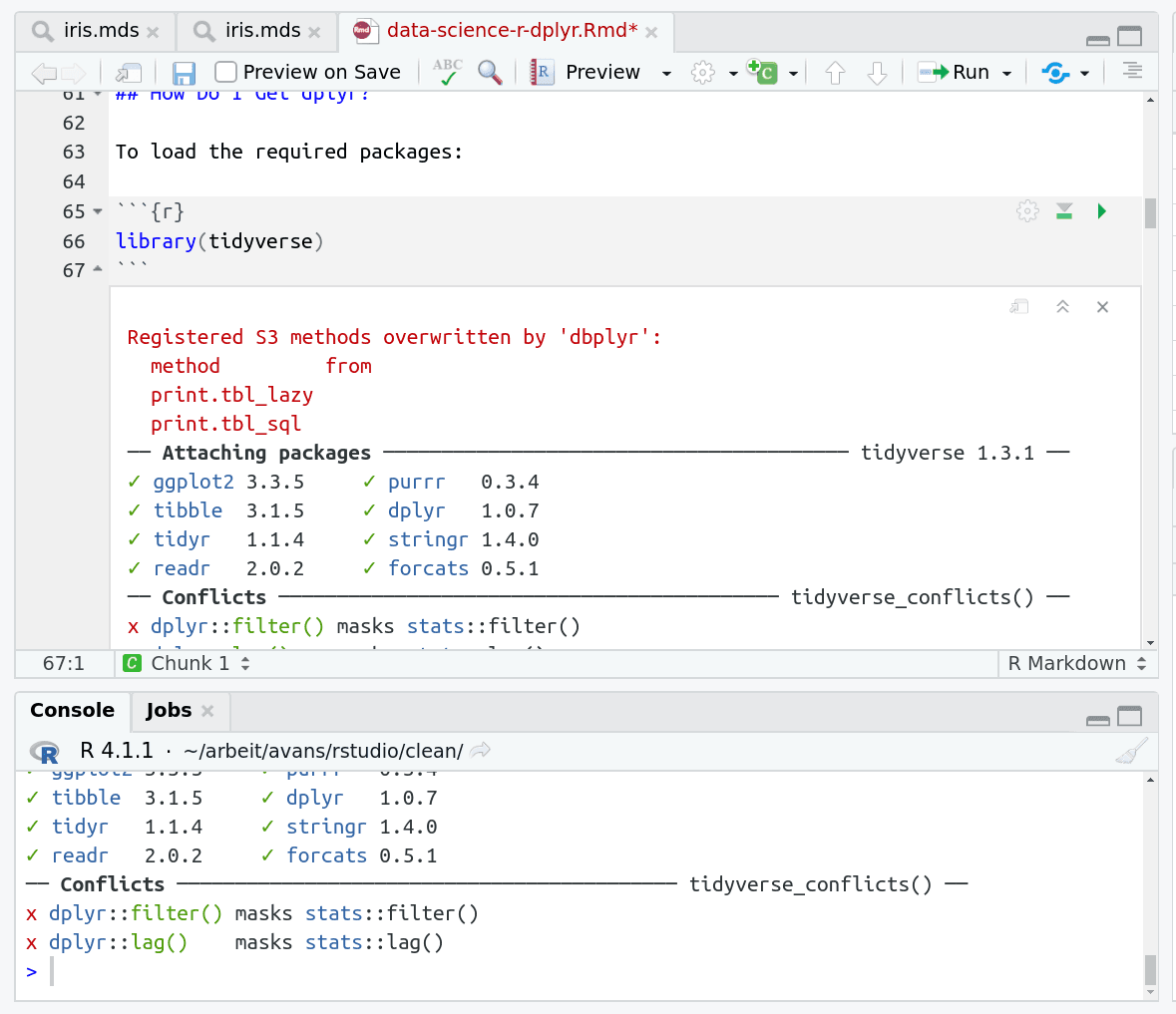One of the best features of RMarkdown documents is that they include a very nice table browser which makes previewing results a lot easier! Instead of needing to use `head` every time to preview the result, you get an interactive table browser for any step which outputs a table.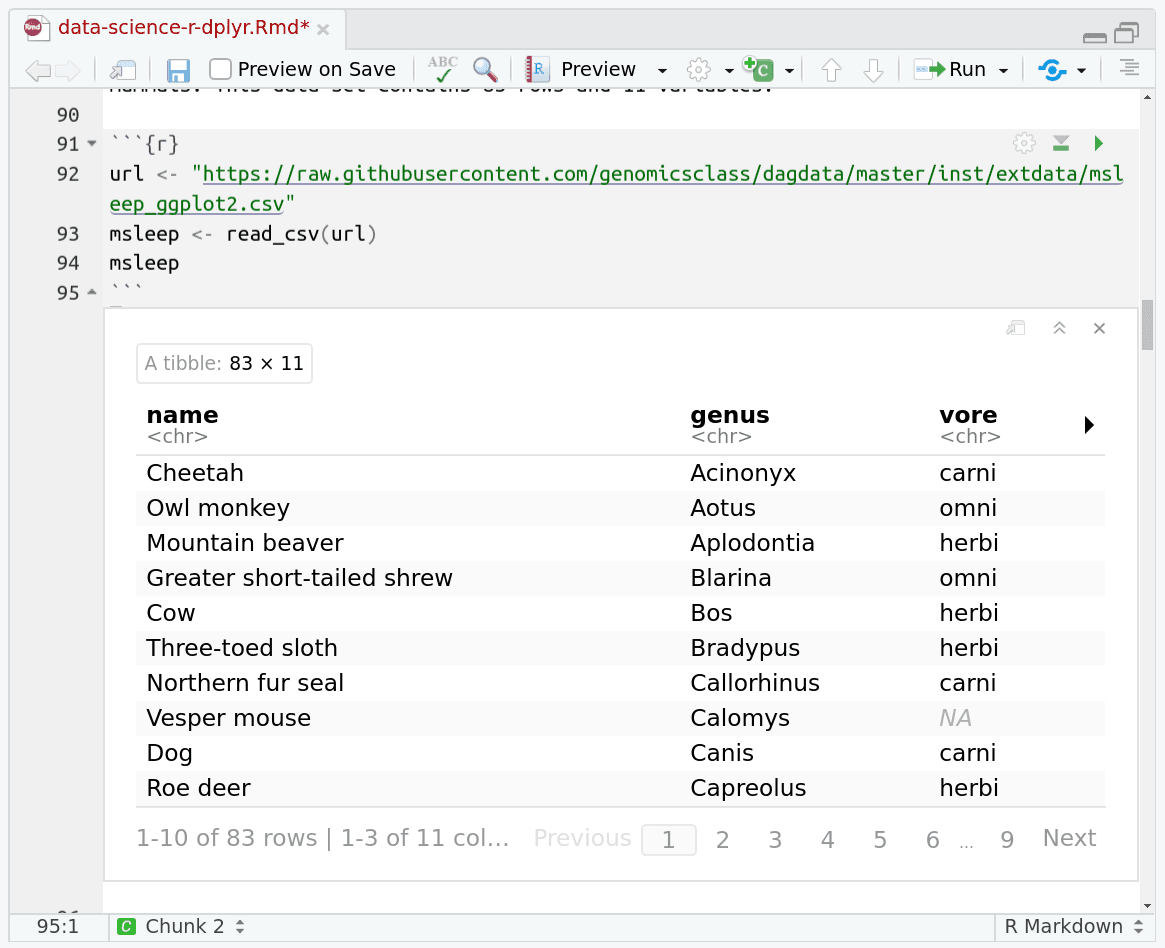In this tutorial, you will learn how to produce scatter plots, boxplots, and time series plots using ggplot. You will also learn how to set universal plot settings, modify the aesthetics of an existing ggplot plots (including axis labels and color), and learn how to facet in ggplot.

Comment

This tutorial is significantly based on Data Carpentry lesson “Data visualization with ggplot2”.

Agenda

In this tutorial, we will cover:

1. Background

# Background

In this tutorial, we will use as our dataset a table with results from the Olympics, from the games in Athens in 1896 until Tokyo in 2020. The objective is to familiarize you with a large number of the most important data visualisation tools in Galaxy. Much like the Olympics, there are many different disciplines (types of operations), and for each operation there are often multiple techniques (tools) available to athletes (data analysts, you) that are great for achieving the goal.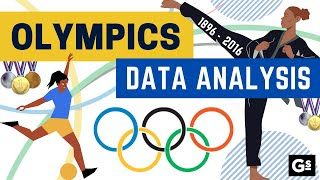We will show you many of these commonly needed visualisation operations, and some examples of how to perform them in R. We also provide many exercises so that you can train your skills and become a data visualisation Olympian!

## Data Visualization with ggplot2

We start by loading the required packages. `ggplot2` is included in the `tidyverse` package.

``````library(tidyverse)
``````

Before we can do any visualisation, we will need some data. Let’s download our table with Olympics results now.

``````olympics <- read_tsv("https://zenodo.org/record/6803028/files/olympics.tsv")
``````
``````View(olympics)
``````
Question
1. What is the format of the file?
2. How is it structured?
3. How many lines are in the file?
4. How many columns?
1. When you expand the `olympics.tsv` dataset in your history (see also screenshot below), you will see `format: tabular`, this is another term for a tab-separated (`tsv`) file.
2. Each row represents an athlete’s participation in an event. If an athlete competes in multiple events, there is a line for each event.
3. 234,522. Look at the bottom of the View or Environment panels to see this number.
4. There are 17 columns in this file. See View or Environment panels.

The data was obtained from Olympedia. The file `olympics.tsv` contains 234,522 rows and 17 columns. Each row corresponds to an individual athlete competing in an individual Olympic event. The columns are:

• athlete_id - Unique number for each athlete
• name - Athlete’s name
• sex - M or F
• birth_year - 4-digit number
• birth_day - e.g. 24 July
• birth_place - town and/or country
• height - In centimeters (or `NA` if data not known)
• weight - In kilograms (or `NA` if data not known)
• team - Team name
• noc - National Olympic Committee 3-letter code
• games - Year and season
• year - Integer
• season - Summer or Winter
• city - Host city
• sport - Sport
• event - Event
• medal - Gold, Silver, Bronze (or `NA` if no medal was won)

We will use this dataset to practice our data visualisation skills in Galaxy.

## Plotting with `ggplot2`

`ggplot2` is a plotting package that provides helpful commands to create complex plots from data in a data frame. It provides a more programmatic interface for specifying what variables to plot, how they are displayed, and general visual properties. Therefore, we only need minimal changes if the underlying data change or if we decide to change from a bar plot to a scatterplot. This helps in creating publication quality plots with minimal amounts of adjustments and tweaking.

`ggplot2` refers to the name of the package itself. When using the package we use the function `ggplot()` to generate the plots, and so references to using the function will be referred to as `ggplot()` and the package as a whole as `ggplot2`

`ggplot2` plots work best with data in the ‘long’ format, i.e., a column for every variable, and a row for every observation. Well-structured data will save you lots of time when making figures with `ggplot2`

ggplot graphics are built layer by layer by adding new elements. Adding layers in this fashion allows for extensive flexibility and customization of plots.

To build a ggplot, we will use the following basic template that can be used for different types of plots:

``````ggplot(data = <DATA>, mapping = aes(<MAPPINGS>)) +  <GEOM_FUNCTION>()
``````

use the `ggplot()` function and bind the plot to a specific data frame using the `data` argument

``````ggplot(data = olympics)
``````
• define an aesthetic mapping (using the aesthetic (`aes`) function), by selecting the variables to be plotted and specifying how to present them in the graph, e.g., as x/y positions or characteristics such as size, shape, color, etc.
``````ggplot(data = olympics, mapping = aes(x = year, y = height))
``````
• add ‘geoms’ – graphical representations of the data in the plot (points, lines, bars). `ggplot2` offers many different geoms; we will use some common ones today, including:

• `geom_point()` for scatter plots, dot plots, etc.
• `geom_boxplot()` for, well, boxplots!
• `geom_line()` for trend lines, time series, etc.

To add a geom to the plot use `+` operator. Because we have two continuous variables, let’s use `geom_point()` first:

``````ggplot(data = olympics, aes(x = year, y = height)) +
geom_point()
``````

The `+` in the `ggplot2` package is particularly useful because it allows you to modify existing `ggplot` objects. This means you can easily set up plot “templates” and conveniently explore different types of plots, so the above plot can also be generated with code like this:

``````# Assign plot to a variable
height_plot <- ggplot(data = olympics,
mapping = aes(x = year, y = height))

# Draw the plot
height_plot +
geom_point()
``````
``````## Create a ggplot and draw it.
height_plot <- ggplot(data = olympics,
aes(x = year, y = height))

height_plot +
geom_point()
``````
• Anything you put in the `ggplot()` function can be seen by any geom layers that you add (i.e., these are universal plot settings). This includes the x- and y-axis you set up in `aes()`.
• You can also specify aesthetics for a given geom independently of the aesthetics defined globally in the `ggplot()` function.
• The `+` sign used to add layers must be placed at the end of each line containing a layer. If, instead, the `+` sign is added in the line before the other layer, `ggplot2` will not add the new layer and will return an error message.
• You may notice that we sometimes reference ‘ggplot2’ and sometimes ‘ggplot’. To clarify, ‘ggplot2’ is the name of the most recent version of the package. However, any time we call the function itself, it’s just called ‘ggplot’.
• The previous version of the `ggplot2` package, called `ggplot`, which also contained the `ggplot()` function is now unsupported and has been removed from CRAN in order to reduce accidental installations and further confusion.
``````# This is the correct syntax for adding layers
height_plot +
geom_point()

# This will not add the new layer and will return an error message
# height_plot
#   + geom_point()
``````
Challenge (optional)

Scatter plots can be useful exploratory tools for small datasets. For data sets with large numbers of observations, such as the `olympics` data set, overplotting of points can be a limitation of scatter plots. One strategy for handling such settings is to use hexagonal binning of observations. The plot space is tessellated into hexagons. Each hexagon is assigned a color based on the number of observations that fall within its boundaries. To use hexagonal binning with `ggplot2`, first install the R package `hexbin` from CRAN:

``````# install.packages("hexbin")
library(hexbin)
``````

Then use the `geom_hex()` function:

``````height_plot +
geom_hex()
``````

What are the relative strengths and weaknesses of a hexagonal bin plot compared to a scatter plot? Examine the above scatter plot and compare it with the hexagonal bin plot that you created.

## Data Cleaning & Calculations

We’ll calculate some new fields to enable us to answer more questions, let’s do that now.

``````olympics <- olympics %>%
mutate(age = year - birth_year) %>%
mutate(weight = as.integer(weight)) %>%
filter(!is.na(weight)) %>%
filter(!is.na(height))
``````

And to speed up future plots, let’s pick three countries and three sports we’re interested in to reduce the amount of data we’ll need to plot:

``````sports = c("Archery", "Judo", "Speed Skating")
# You can change this to any of:
# c("Alpine Skiing", "Archery", "Art Competitions", "Artistic Gymnastics", "Artistic Swimming", "Athletics", "Badminton", "Baseball", "Basketball", "Biathlon", "Bobsleigh", "Bowling", "Boxing", "Canoe Marathon", "Canoe Slalom", "Canoe Sprint", "Cross Country Skiing", "Cycling BMX Freestyle", "Cycling BMX Racing", "Cycling Mountain Bike", "Cycling Road", "Cycling Track", "Diving", "Dogsled Racing", "Equestrian Dressage", "Equestrian Eventing", "Equestrian Jumping", "Fencing", "Figure Skating", "Freestyle Skiing", "Golf", "Handball", "Hockey", "Judo", "Karate", "Luge", "Marathon Swimming", "Military Ski Patrol", "Modern Pentathlon", "Nordic Combined", "Rhythmic Gymnastics", "Rowing", "Rugby", "Sailing", "Shooting", "Short Track Speed Skating", "Skateboarding", "Skeleton", "Ski Jumping", "Snowboarding", "Speed Skating", "Speed Skiing", "Surfing", "Swimming", "Table Tennis", "Taekwondo", "Tennis", "Trampolining", "Triathlon", "Tug-Of-War", "Volleyball", "Water Polo", "Weightlifting", "Winter Pentathlon", "Wrestling", "Wushu")

countries = c("NED", "USA", "CHN")

olympics_small <- olympics %>% filter(sport %in% sports) %>% filter(noc %in% countries)
``````

Building plots with `ggplot2` is typically an iterative process. We start by defining the dataset we’ll use, lay out the axes, and choose a geom:

``````ggplot(olympics_small, aes(x=age, y=sport)) +
geom_point()
``````

Then, we start modifying this plot to extract more information from it. For instance, we can add transparency (`alpha`) to avoid overplotting:

``````ggplot(olympics_small, aes(x=age, y=sport)) +
geom_point(alpha = 0.1)
``````

We can also add colors for all the points:

``````ggplot(olympics_small, aes(x=age, y=sport)) +
geom_point(alpha = 0.1, color = "blue")
``````

Or to color each species in the plot differently, you could use a vector as an input to the argument color. `ggplot2` will provide a different color corresponding to different values in the vector. Here is an example where we color with `species_id`:

``````ggplot(olympics_small, aes(x=age, y=sport)) +
geom_point(alpha = 0.1, aes(color = sex))
``````
Challenge

Use what you just learned to create a scatter plot of `height` over `sport` with the plot types showing the season in different colors. Is this a good way to show this type of data?

``````ggplot(data = olympics,
mapping = aes(x = height, y = sport)) +
geom_point(aes(color = season))
``````

## Boxplot

We can use boxplots to visualize the distribution of height within each sport:

``````ggplot(data = olympics_small, mapping = aes(x = height, y = sport)) +
geom_boxplot()
``````

But this is a bit boring with all three merged together, so let’s colour by NOC.

``````ggplot(olympics_small, aes(x = height, y = sport, fill=noc)) +
geom_boxplot()
``````

By adding points to the boxplot, we can have a better idea of the number of measurements and of their distribution. Because the boxplot will show the outliers by default these points will be plotted twice – by `geom_boxplot` and `geom_jitter`. To avoid this we must specify that no outliers should be added to the boxplot by specifying `outlier.shape = NA`.

``````ggplot(olympics_small, aes(x = height, y = sport, fill=noc)) +
geom_boxplot(outlier.shape = NA) +
geom_jitter(alpha = 0.3, color = "orange")
``````

Notice how the boxplot layer is behind the jitter layer? What do you need to change in the code to put the boxplot in front of the points such that it’s not hidden?

Challenge

Boxplots are useful summaries, but hide the shape of the distribution. For example, if there is a bimodal distribution, it would not be observed with a boxplot. An alternative to the boxplot is the violin plot (sometimes known as a beanplot), where the shape (of the density of points) is drawn.

• Replace the box plot with a violin plot; see `geom_violin()`.
``````ggplot(olympics_small, aes(x = height, y = sport)) +
geom_jitter(alpha = 0.3, color = "orange") +
geom_violin()
``````

In many types of data, it is important to consider the scale of the observations. For example, it may be worth changing the scale of the axis to better distribute the observations in the space of the plot. Changing the scale of the axes is done similarly to adding/modifying other components (i.e., by incrementally adding commands). Try making these modifications:

• Represent height on the `log_10` scale; see `scale_y_log10()` and `scale_x_log10()`.
``````ggplot(olympics_small, aes(x = height, y = sport)) +
scale_x_log10() +
geom_jitter(alpha = 0.3, color = "orange") +
geom_boxplot(outlier.shape = NA)
``````

So far, we’ve looked at the distribution of height within specific sports. Try making a new plot to explore the distribution of another variable within each sport!

Create boxplot for `age` by sport. Overlay the boxplot layer on a jitter layer to show actual measurements.

``````ggplot(olympics_small, aes(x = age, y = sport)) +
geom_jitter(alpha = 0.3, color = "orange") +
geom_boxplot(outlier.shape = NA)
``````

Add color to the data points on your boxplot according to the year from which the sample was taken (`year`).

Hint: Check the class for `year`. Consider changing the class of `year` from integer to factor. Why does this change how R makes the graph?

``````ggplot(olympics_small, aes(x = age, y = sport)) +
geom_jitter(alpha = 0.3, aes(color=year)) +
geom_boxplot(outlier.shape = NA)

# As a factor:
ggplot(olympics_small, aes(x = age, y = sport)) +
geom_jitter(alpha = 0.3, aes(color=as.factor(year))) +
geom_boxplot(outlier.shape = NA)
``````

## Plotting time series data

Let’s calculate number of participants per year for each games. First we need to group the data and count records within each group:

``````yearly_counts <- olympics %>% count(year, season)
``````

Timelapse data can be visualized as a line plot with years on the x-axis and counts on the y-axis:

``````ggplot(data = yearly_counts, aes(x = year, y = n)) +
geom_line()
``````

Unfortunately, this does not work well because our data is quite sparse, datapoints only ever 2 or 4 years. Let’s instead use a box plot

``````ggplot(data = yearly_counts, aes(x = year, y = n)) +
geom_col()
``````

We can’t use `geom_box()` here, instead we should use `geom_col()` as our data is already aggregated.

We need to tell ggplot to draw a line for each season by modifying the aesthetic function to include `group = season`:

``````ggplot(data = yearly_counts, aes(x = year, y = n, group = season)) +
geom_col()
``````

We will be able to distinguish season in the plot if we add colors (using `color` or `fill` also automatically groups the data:

``````ggplot(data = yearly_counts, aes(x = year, y = n, fill = season)) +
geom_col()
``````

If you want the histograms to be side-by-side, we can do that with the “dodge” positioning:

``````ggplot(data = yearly_counts, aes(x = year, y = n, fill = season)) +
geom_col(position = "dodge")
``````

## Integrating the pipe operator with ggplot2

In the previous lesson, we saw how to use the pipe operator `%>%` to use different functions in a sequence and create a coherent workflow. We can also use the pipe operator to pass the `data` argument to the `ggplot()` function. The hard part is to remember that to build your ggplot, you need to use `+` and not `%>%`.

``````yearly_counts %>%
ggplot(aes(x = year, y = n, fill = season)) +
geom_col()
``````

The pipe operator can also be used to link data manipulation with consequent data visualization.

``````yearly_counts_graph <- olympics %>%
count(year, season) %>%
ggplot(mapping = aes(x = year, y = n, fill = season)) +
geom_col()

yearly_counts_graph
``````

## Faceting

`ggplot` has a special technique called faceting that allows the user to split one plot into multiple plots based on a factor included in the dataset. We will use it to make a time series plot for each season separately:

``````yearly_counts %>%
ggplot(aes(x = year, y = n, fill = season)) +
geom_col() + facet_wrap(facets=vars(season))
``````

Now we would like to split the line in each plot by the sex of each individual, sport, and their medal placement. To do that we need to make counts in the data frame grouped by `year`, `season`, `sport`, `medal`, and `noc`.

``````year_detail_counts <- olympics_small %>%
count(year, season, sport, medal, noc)
``````

We can now make the faceted plot by splitting further by medal using `color` (within a single plot), and per NOC:

``````ggplot(data = year_detail_counts, mapping = aes(x = year, y = n, color = medal)) +
geom_line() +
facet_wrap(facets =  vars(noc))
``````

We can also facet both by NOC and sport:

``````ggplot(data = year_detail_counts, mapping = aes(x = year, y = n, color = medal)) +
geom_line() +
facet_grid(rows = vars(noc), cols =  vars(sport))
``````

You can also organise the panels only by rows (or only by columns):

``````# One column, facet by rows
ggplot(data = year_detail_counts, mapping = aes(x = year, y = n, color = medal)) +
geom_line() +
facet_grid(rows = vars(noc))
``````
``````# One row, facet by column
ggplot(data = year_detail_counts, mapping = aes(x = year, y = n, color = medal)) +
geom_line() +
facet_grid(cols = vars(noc))
``````

`ggplot2` before version 3.0.0 used formulas to specify how plots are faceted. If you encounter `facet_grid`/`wrap(...)` code containing `~`, please read https://ggplot2.tidyverse.org/news/#tidy-evaluation.

## `ggplot2` themes

Usually plots with white background look more readable when printed. Every single component of a `ggplot` graph can be customized using the generic `theme()` function, as we will see below. However, there are pre-loaded themes available that change the overall appearance of the graph without much effort.

For example, we can change our previous graph to have a simpler white background using the `theme_bw()` function:

``````ggplot(data = year_detail_counts, mapping = aes(x = year, y = n, color = medal))  +
geom_line() +
facet_grid(rows = vars(noc), cols =  vars(sport)) +
theme_bw()
``````

In addition to `theme_bw()`, which changes the plot background to white, `ggplot2` comes with several other themes which can be useful to quickly change the look of your visualization. The complete list of themes is documented in the ggthemes reference. `theme_minimal()` and `theme_light()` are popular, and `theme_void()` can be useful as a starting point to create a new hand-crafted theme.

The ggthemes package provides a wide variety of options.

Challenge

Use what you just learned to create a plot the relationship between height and weight, of participants, broken down by NOC and Sport.

``````ggplot(data = olympics_small, mapping = aes(x = height, y = weight, color = medal)) +
geom_point() +
facet_grid(rows = vars(noc), cols = vars(sport))
``````

## Customization

Take a look at the `ggplot2` cheat sheet, and think of ways you could improve the plot.

Now, let’s change names of axes to something more informative than ‘year’ and ‘n’ and add a title to the figure:

``````ggplot(data = year_detail_counts, mapping = aes(x = year, y = n, fill = medal))  +
geom_col() +
facet_grid(rows = vars(noc), cols =  vars(sport)) +
labs(title = "Participants and medals over the years",
x = "Year",
y = "Number of individuals") +
theme_bw()
``````

The axes have more informative names, but their readability can be improved by increasing the font size. This can be done with the generic `theme()` function:

``````ggplot(data = year_detail_counts, mapping = aes(x = year, y = n, fill = medal))  +
geom_col() +
facet_grid(rows = vars(noc), cols =  vars(sport)) +
labs(title = "Participants and medals over the years",
x = "Year",
y = "Number of individuals") +
theme_bw()
theme(text=element_text(size = 16))
``````

Note that it is also possible to change the fonts of your plots. If you are on Windows, you may have to install the `extrafont` package, and follow the instructions included in the README for this package.

After our manipulations, you may notice that the values on the x-axis are still not properly readable. Let’s change the orientation of the labels and adjust them vertically and horizontally so they don’t overlap. You can use a 90 degree angle, or experiment to find the appropriate angle for diagonally oriented labels. We can also modify the facet label text (`strip.text`) to italicize the genus names:

``````ggplot(data = year_detail_counts, mapping = aes(x = year, y = n, fill = medal))  +
geom_col() +
facet_grid(rows = vars(noc), cols =  vars(sport)) +
labs(title = "Participants and medals over the years",
x = "Year",
y = "Number of individuals") +
theme_bw()
theme(axis.text.x = element_text(colour = "grey20", size = 12, angle = 90, hjust = 0.5, vjust = 0.5),
axis.text.y = element_text(colour = "grey20", size = 12),
strip.text = element_text(face = "italic"),
text = element_text(size = 16))
``````

If you like the changes you created better than the default theme, you can save them as an object to be able to easily apply them to other plots you may create:

``````grey_theme <- theme(axis.text.x = element_text(colour="grey20", size = 12,
angle = 90, hjust = 0.5,
vjust = 0.5),
axis.text.y = element_text(colour = "grey20", size = 12),
text=element_text(size = 16))

ggplot(data = year_detail_counts, mapping = aes(x = year, y = n, fill = medal))  +
geom_col() +
facet_grid(rows = vars(noc), cols =  vars(sport)) +
grey_theme
``````
Challenge

With all of this information in hand, please take another five minutes to either improve one of the plots generated in this exercise or create a beautiful graph of your own. Use the RStudio `ggplot2` cheat sheet for inspiration.

Here are some ideas:

• See if you can change the plot type to another plot
• Can you find a way to change the name of the legend? What about its labels?
• Try using a different color palette (see https://r-graphics.org/chapter-colors).

This optional exercise currently lacks solutions. If you have them, please feel free to contribute suggestions here :)

## Arranging plots

Faceting is a great tool for splitting one plot into multiple plots, but sometimes you may want to produce a single figure that contains multiple plots using different variables or even different data frames. The `patchwork` package allows us to combine separate ggplots into a single figure while keeping everything aligned properly. Like most R packages, we can install `patchwork` from CRAN, the R package repository, if it isn’t already available:

``````# install.packages("patchwork")
``````

After you have loaded the `patchwork` package you can use `+` to place plots next to each other, `/` to arrange them vertically, and `plot_layout()` to determine how much space each plot uses:

``````library(patchwork)

plot_weight <- olympics_small %>% ggplot(aes(x=noc, y=weight)) +
geom_boxplot() +
labs(x = "NOC", y = expression(log(Weight))) +
scale_y_log10()

plot_height <- olympics_small %>% ggplot(aes(x=noc, y=height)) +
geom_boxplot() +
labs(x = "NOC", y = expression(log(Height))) +
scale_y_log10()

plot_weight / plot_height + plot_layout(heights = c(3, 2))
``````

You can also use parentheses `()` to create more complex layouts. There are many useful examples on the patchwork website

## Exporting plots

After creating your plot, you can save it to a file in your favorite format. The Export tab in the Plot pane in RStudio will save your plots at low resolution, which will not be accepted by many journals and will not scale well for posters. The `ggplot2` extensions website provides a list of packages that extend the capabilities of `ggplot2`, including additional themes.

Instead, use the `ggsave()` function, which allows you to easily change the dimension and resolution of your plot by adjusting the appropriate arguments (`width`, `height` and `dpi`):

``````my_plot <- year_detail_counts %>% ggplot(aes(x = year, y = n, fill = medal))  +
geom_col() +
facet_grid(rows = vars(noc), cols =  vars(sport)) +
labs(title = "Participants and medals over the years",
x = "Year",
y = "Number of individuals") +
theme_bw()
theme(axis.text.x = element_text(colour = "grey20", size = 12, angle = 90, hjust = 0.5, vjust = 0.5),
axis.text.y = element_text(colour = "grey20", size = 12),
strip.text = element_text(face = "italic"),
text = element_text(size = 16))

ggsave("name_of_file.png", my_plot, width = 15, height = 10)

## This also works for plots combined with patchwork
plot_combined <- plot_weight / plot_height + plot_layout(heights = c(3, 2))
ggsave("plot_combined.png", plot_combined, width = 10, dpi = 300)
``````

Note: The parameters `width` and `height` also determine the font size in the saved plot.

``````### Final plotting challenge:
##  With all of this information in hand, please take another five
##  minutes to either improve one of the plots generated in this
##  exercise or create a beautiful graph of your own. Use the RStudio
##  ggplot2 cheat sheet for inspiration: